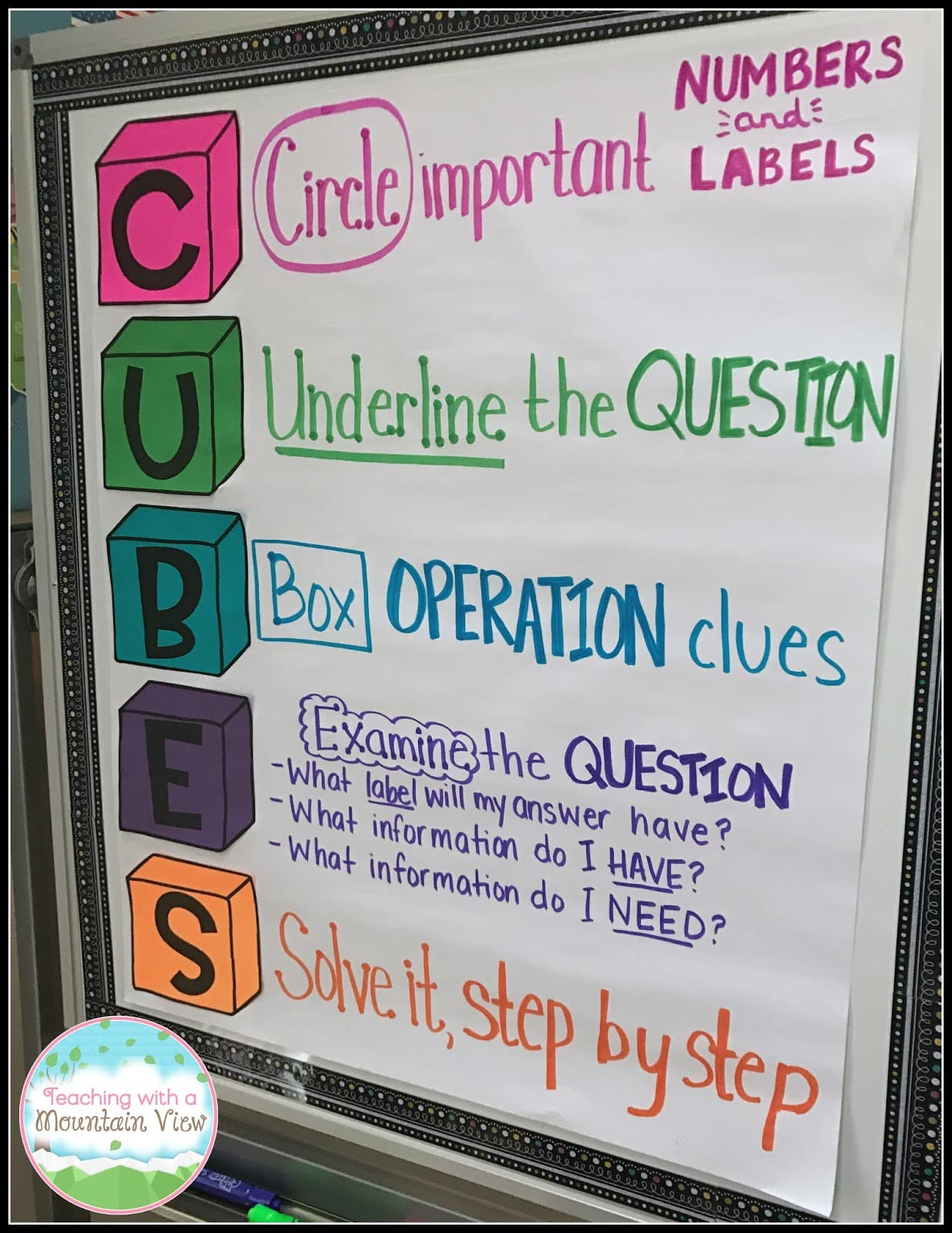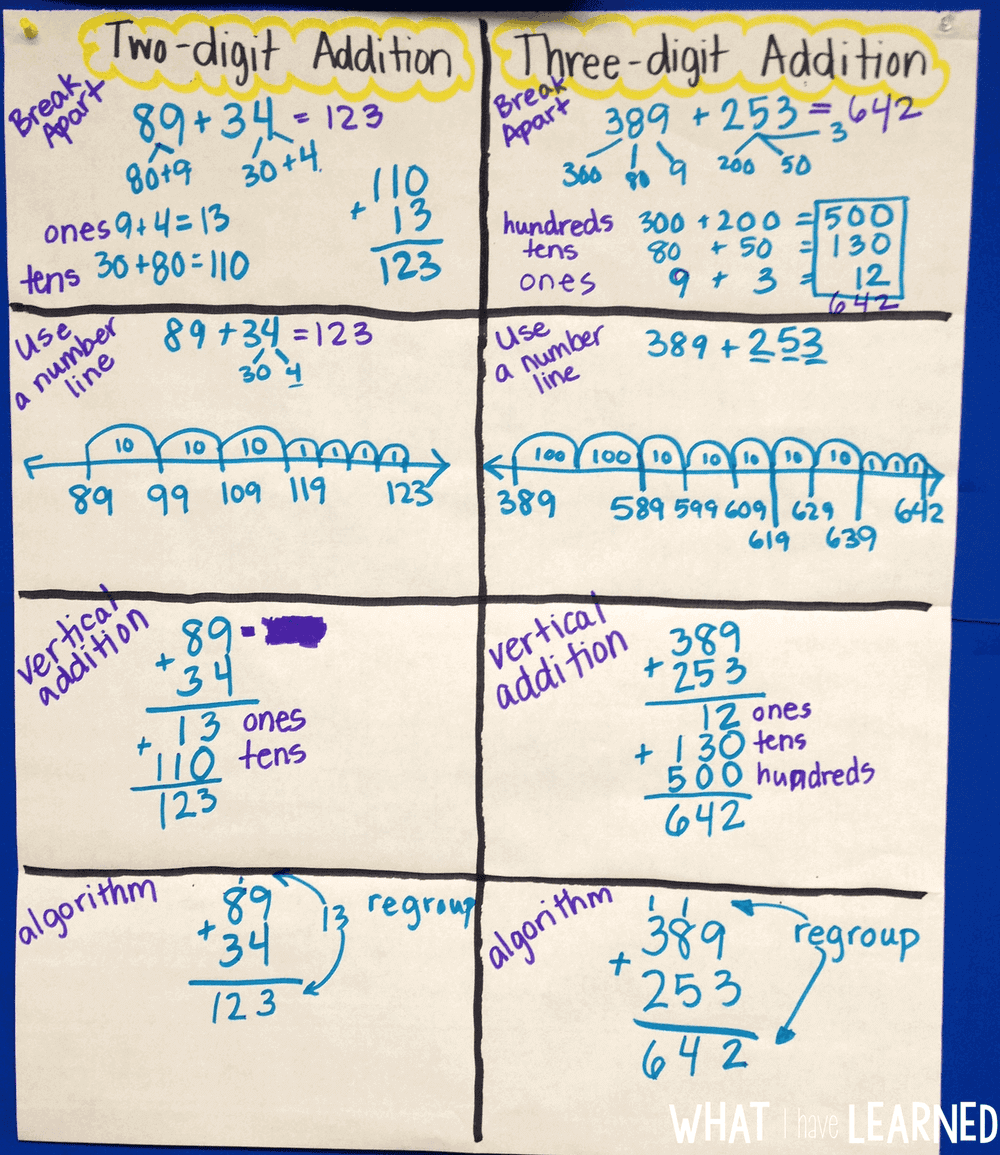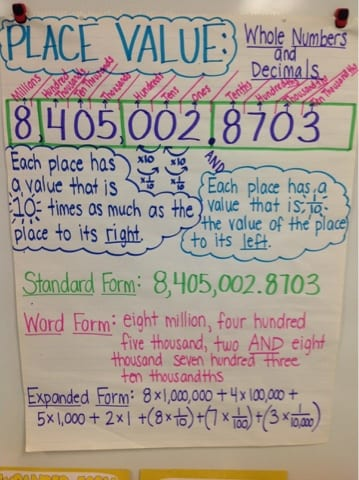• The following resources will help your scholar complete his/her MATH packet.  The worksheets included in this packet include all second grade review standards, except for the last page which is our first third grade topic- Rounding Using Place Value.

SECOND GRADE STANDARDS that are covered:

MGSE2.NBT.1 Understand that the three digits of a three-digit number represent amounts of hundreds, tens, and ones; e.g., 706 equals 7 hundreds, 0 tens, and 6 ones. a. 100 can be thought of as a bundle of ten tens — called a ―hundred. b. The numbers 100, 200, 300, 400, 500, 600, 700, 800, 900 refer to one, two, three, four, five, six, seven, eight, or nine hundreds (and 0 tens and 0 ones).

MGSE2.NBT.2 Count within 1000; skip-count by 5s, 10s, and 100s.

MGSE2.NBT.3 Read and write numbers to 1000 using base-ten numerals, number names, and expanded form.

MGSE2.NBT.4 Compare two three-digit numbers based on meanings of the hundreds, tens, and ones digits, using >, =, and < symbols to record the results of comparisons.

MGSE2.OA.1 Use addition and subtraction within 100 to solve one and two step word problems by using drawings and equations with a symbol for the unknown number to represent the problem. Problems include contexts that involve adding to, taking from, putting together/taking apart (part/part/whole) and comparing with unknowns in all positions.

MGSE2.NBT.7 Add and subtract within 1000, using concrete models or drawings and strategies based on place value, properties of operations, and/or the relationship between addition and subtraction; relate the strategy to a written method.

THIRD GRADE STANDARD that is covered:

MGSE3.NBT.1 Use place value understanding to round whole numbers to the nearest 10 or 100.

ANCHOR CHARTS: